login  home  contents  what's new  discussion  bug reports help  links  subscribe  changes  refresh  edit

 Submitted by : (unknown) at: 2007-11-17T21:54:03-08:00 (15 years ago) Name : Axiom Version : default friCAS-20090114 Axiom-20050901 OpenAxiom-20091012 OpenAxiom-20110220 OpenAxiom-Release-141 Category : Axiom Aldor Interface Axiom Compiler Axiom Library Axiom Interpreter Axiom Documentation Axiom User Interface building Axiom from source lisp system MathAction Doyen CD Reduce Axiom on Windows Axiom on Linux Severity : critical serious normal minor wishlist Status : open closed rejected not reproducible fix proposed fixed somewhere duplicate need more info Optional subject :   Optional comment :

Only one of these answers is correct:

fricas
(1) -> eq1:=%pi/2-asin(n/2)=asin(n)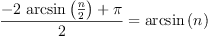(1)
Type: Equation(Expression(Integer))
fricas
s:=solve(eq1,n)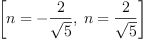(2)
Type: List(Equation(Expression(Integer)))
fricas
-- repeating is ok
s:=solve(eq1,n)(3)
Type: List(Equation(Expression(Integer)))
fricas
subst(eq1,s.1)::Equation Expression Float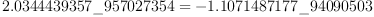(4)
Type: Equation(Expression(Float))
fricas
subst(eq1,s.2)::Equation Expression Float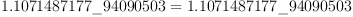(5)
Type: Equation(Expression(Float))

We should expect the same result from:

fricas
)clear all
All user variables and function definitions have been cleared.
eq1:=%pi/2-asin(n/2)=asin(n)(6)
Type: Equation(Expression(Integer))
fricas
s:=solve(eq1,n)(7)
Type: List(Equation(Expression(Integer)))
fricas
-- repeating is not ok!
s:=solve(eq1,n)(8)
Type: List(Equation(Expression(Integer)))
fricas
subst(eq1,s.1)::Equation Expression Float(9)
Type: Equation(Expression(Float))
fricas
subst(eq1,s.2)::Equation Expression Float(10)
Type: Equation(Expression(Float))
fricas
subst(eq1,s.3)::Equation Expression Float
>> Error detected within library code:
index out of range

But now there are 4 results for the same equation!

Why Complex Float? --Bill Page, Wed, 09 Mar 2005 23:14:48 -0600 reply
Why do I need to use Complex Float in order to evaluate the numeric value of these expression? Just Float will not work
fricas
asin(1/2)::Float
Cannot convert the value from type Expression(Integer) to Float .

From: wyscc, Thur, 10 Mar 2005 08:16:00

Of course you don't, from a mathematical view point, and the problem is apparently the Interpreter needs help. If you put the argument into Float or the expression into Expression Float, Axiom will oblige.

fricas
asin(1/2::Float)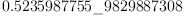(11)
Type: Float
fricas
asin(1/2)::Expression Float(12)
Type: Expression(Float)

But in fact, even coercion to Complex Float won't always work.

fricas
asin(%i/2)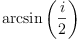(13)
Type: Expression(Complex(Integer))
fricas
asin(%i/2)::Complex Float
Cannot convert the value from type Expression(Complex(Integer)) to
Complex(Float) .

There is no modemap from Expression Integer to Complex Float (Use hyperdoc Browse, Selectable to search, with wild character in the name field). This is reasonable since it is not possible in general to evaluate numerically a symbolic expression. I believe the Interpreter actually tries this:

fricas
asin(1/2)$Float(14) Type: Float fricas asin(1/2)$(Complex Float)(15)
Type: Complex(Float)

which succeed in both cases because asin has modemaps in those domains. Exactly why it was able to change a non-existing coercion in one case but not the other is unclear, but this seems to be because the Interpreter code is not as categorical as the compiler code and these smart'' coercions may be done case by case. But even this reasoning has problem:

fricas
asin(%i/2::Complex Float)
easiest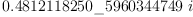(16)
Type: Complex(Float)
fricas
asin(%i/2)::Expression Complex Float::Complex Float -- harder(17)
Type: Complex(Float)
fricas
asin(%i/2)$(Complex Float) -- doesn't work There are 1 exposed and 6 unexposed library operations named asin having 1 argument(s) but none was determined to be applicable. Use HyperDoc Browse, or issue )display op asin to learn more about the available operations. Perhaps package-calling the operation or using coercions on the arguments will allow you to apply the operation. Cannot find a definition or applicable library operation named asin with argument type(s) Fraction(Complex(Integer)) Perhaps you should use "@" to indicate the required return type, or "$" to specify which version of the function you need.

Ah, so subtle are the Axiom types! --Bill Page, Thu, 10 Mar 2005 09:50:00 -0600 reply
William,

Thank you for the explanation. Now I "get it". The kind of coercion that I really wanted to do was like this:

  sin(1)::Expression Float


This is taking something from Expression Integer to Expression Float which always works even for:

fricas
sin(x)::Expression Float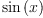(18)
Type: Expression(Float)

But when x converts to Float then the whole expression can be displayed like Float (even though it remains Expression Float!). In the coercion we are just changing the ground type of the Expression. In fact it can be converted to Float by the function ground.

fricas
ground(sin(1)::Expression Float)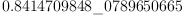(19)
Type: Float

Or just

fricas
sin(1)::Expression Float::Float(20)
Type: Float

Perhaps a function groundIfCan would be nice :)

But in general the interpreter should not be expected know that such a chain of coercions is possible. Right

Neat and very general. Its the same for all trig, exp, log, etc. functions.

So now I also agree that the coercion to Complex Float does not make sense. Notice that the following error messages should be the same:

fricas
log(10.0*q)::Float
Cannot convert the value from type Expression(Float) to Float .

But the Complex Float domain is doing something extra.

If this is because of the interpreter then I think it is trying too hard and as a result it makes it difficult to explain this behaviour to the novice user. In this case I would prefer the interpretation to be more categorical and consistent so that we can explain this subtly from the very beginning.

Bill Page.

property change --Bill Page, Thu, 10 Mar 2005 22:32:58 -0600 reply
From: wyscc, Fri, 11 Mar 2005 00:37:00
Perhaps a function groundIfCan would be nice :)

The origin implementation of ground in Expression is from FunctionSpace (according to Hyperdoc) and may give an error if the argument is not from the ground domain. There is a function ground? which does the test. A more common (and indeed more general) function is retractIfCan, which would give "failed" if the retraction cannot be done. There are 8 modemaps for retractIfCan in Expression Float and you can retract to Algebraic Number, Float, Fraction Integer, Fraction Polynomial Float, Integer, Kernel Expression Float, Polynomial Float and Symbol. As far as MathAction goes, I would prefer "failed" rather than an error, because an error stops the running of the rest of Axiom script block.

Cannot compute the numerical value of a non-constant expression
But the Complex Float domain is doing something extra.

The issues here are two: The first two errors are modemap problems. The last one is an anticipated error message from algebra code. I suspect that the Interpreter did not try to find numeric in the first instance (it should), did not find any modemap from POLY FLOAT -> COMPLEX INT (there are none, which makes sense), but found complexNumeric in the last. In the first one, numeric.o is not loaded because the Interpreter somehow is not instructed to look for numeric. Even after numeric.o is loaded, the situation is the same: the Interpreter stops after locating log: EXPR FLOAT ->EXPR FLOAT. In the last case, the Interpreter loads  numeric.o if it is not there. So it would seem that it is a dependency problem during compiling (which presumably provides the database to the Interpreter).

fricas
numeric(log(10.0*q))
>> Error detected within library code:
Can only compute the numerical value of a constant, real-valued Expression

So this treatment agrees with:

fricas
complexNumeric(log(10.0*q))
>> Error detected within library code:
Cannot compute the numerical value of a non-constant expression

which has the same output as log(10.0*q)::Complex Float

By the way, I think this discussion (the second half, involving conversion to Float) should be separated into a new page. Perhaps under a title like "Numerical Type Conversion".

I still have no clue why after a )clear all, the second solve behave the way it did. I have verified that it occurs fairly consistently, even in the NAG version. (It occurred even if I had never run the first eq1, solve, solve before the )clear all if I was working in some session already. But it occurred consistently if I started with a new Axiom window and followed the script.)

Status: open => fixed somewhere

 Subject:   Be Bold !! ( 14 subscribers )